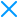# Order

The `order` directive allows you to enable transformations in a specific block or statement and also in a specific order, opposed to `enable` that enables transformations in a random order.

Consider the following contrived example:

```// @jscrambler order dotToBracketNotation, numberToString, stringEncoding
map.moveTo(250, 0)
// @jscrambler order dotToBracketNotation, stringEncoding, numberToString
map.moveTo(250, 0)
// @jscrambler order stringEncoding, numberToString, dotToBracketNotation
map.moveTo(250, 0)```

The resulting code would look something like this:

```map[m.j(2)](+m.p(1), m.r(3) * 1)
map[m.l(4)](1 * '250', '0' | 0)
map['moveTo'](1 * '250', '0' | 0)```

In the first statement, the transformations were combined in a way that allowed String Encoding to target the identifier `moveTo` and the numeric arguments `0` and `250`. On the second statement we have changed the order so String Encoding would happen before Number to String. This way String Encoding didn't target the numeric arguments of the function `map.moveTo`, because they haven't been transformed to strings at the time. The last statement is the worst example because the selected order didn't maximize the synergies between the transformations. Therefore, when String Encoding was called there were no strings to obfuscate.

Imagine you want to set a specific order for the whole JavaScript source code. To do this you can use the `global` modifier.

```// @jscrambler global order dotToBracketNotation, numberToString, stringEncoding
map.moveTo(250, 0)
map.moveTo(0, 250)
map.moveTo(250, 250)```
```map[m.l(6)](m.j(1) | 0, +m.k(3));
map[m.g(4)](+m.f(3), m.l(8) * 1);
map[m.c(4)](1 * m.h(1), m.i(8) - 0);```

You can also repeat transformations. Take a look at the following example:

```// @jscrambler order dotToBracketNotation, numberToString, stringEncoding, numberToString, stringEncoding
map.moveTo(0, 0)```

By repeating Number to String and String Encoding a second time we have transformed the numeric arguments produced by the first String Encoding and encoded all strings a second time therefore producing this code:

``map[m.e(+m.f(1))](m.j(+m.o(2)) * (m.n(0) - 0), +m.g(m.i(3) | 0));``

However, the number of times you can repeat the same transformation is limited. You can only repeat the same transformation up to 3 times. There are some exceptions to this, where transformations can't be repeated, meaning that they can only be used once per node. The transformations that can only be used once are:

`Order` can also be inherited so if you define a specific order for a block, any inner statement and block will inherit it and combine it with any directive defined at that point.

Consider the following example with made up transformations to make it easier to understand how `order` inheritance works:

```// @jscrambler order A, B, C
function foo () {
// @jscrambler order D, E
function bar () {}
// @jscrambler order A, A
function baz () {}
// @jscrambler order B
function qux () {}
}```

Functions `bar`, `baz` and `qux`, inherit from function `foo`:

```// [A, B, C]
function foo () {
// [D, E, A, B, C]
function bar () {}
// [A, A, B, C]
function baz () {}
// [B, A, C]
function qux () {}
}```

Note that the first transformations to be applied are the ones respectively closer to `bar`, `baz`, and `qux`. Also if there are any matching transformation, those are merged in pairs so inheriting a transformation `A` when the same transformation `A` is defined locally, won't apply the transformation twice. For `baz`, transformation `A` has been applied only two times because there was one matching `A` with the order inherited from `foo` that was merged. For `qux`, transformation `B` is applied first (and merged) and then the order inherited from `foo` is applied.